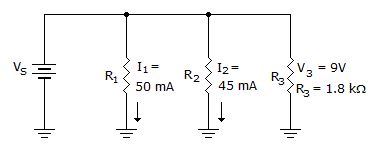# Electronics - Parallel Circuits - Discussion

### Discussion :: Parallel Circuits - General Questions (Q.No.14)

14.What does VS equal in the given circuit?

 [A]. 0 V [B]. 9 V [C]. 27 V [D]. More information is needed to find VS.

Explanation:

No answer description available for this question.

 Seshendra said: (Nov 11, 2010) voltage across the parallel resistors are equal.... So votage Vs equals to the voltage across R3 resistor...

 Chaitu said: (Mar 7, 2011) Polarity information is missing. So the ans may be -9v also.

 Sandeep Kumar said: (Jun 24, 2011) Because voltage is same in parallel circuit and current is same in series circuit.

 Pramod said: (May 12, 2013) I3 = V3/R3 = 9V/1.8KOhm = 5mA. V = I3R3 = 5*1.8 = 9V. Therefore Vs = 9V.

 D Durgaprasad said: (Sep 24, 2013) In parallel circuit the voltage remains constant, so Vs is also 9v.

 Suraj said: (Jan 14, 2015) Voltage drop is equal in each branches. So v3 = vs = 9.

 Mpavankumar said: (May 20, 2015) From the parallel connection same voltage in each branch that is why the answer is 9v.

 Isr said: (Feb 28, 2016) Voltage divides in series and current in parallel.

 Mukesh Kumar said: (Aug 15, 2016) Voltage across parallel combination is always same.

 Krish said: (Sep 10, 2016) Voltage is parallel. V3 = 9. So Vs also 9 V.

 Yogesh Girase said: (Mar 6, 2017) In parallel, the voltage is same. Vs = V1 = V2 = V3.

 Rekha said: (Jan 31, 2018) Hello @Chaitu. Ground means -ve know, so, the answer is +9v.

 Ashpak- Sande said: (Nov 16, 2018) In the parallel. The Ckt voltage is same vs= v1=v2=v3=vn.